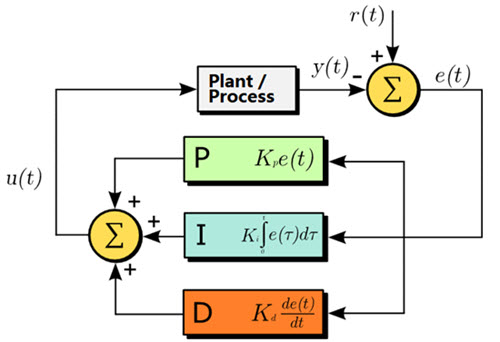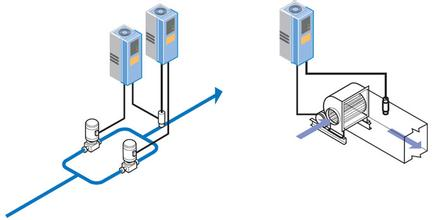# What is PID Control Algorithm

In practical applications of engineering, PID control algorithm is the most widely used algorithm. The following passage is the specific introduction of what is PID control algorithm.

In practical applications of engineering, PID control algorithm is the most widely used algorithm. The following passage is the specific introduction of what is PID control algorithm.

### What is the Work Principle of PID Control Algorithm?

The most widely used regulator control law of proportional, integral and differential control is referred to as PID control algorithm, also known as PID control or PID regulation. PID controllers have come out with an history of nearly 70 years. The simple structure, good stability and reliable performance have become one of the major industrial control technology. When the structure and parameters of the controlled object are not fully given, the structure and parameters of the system controller must be relied on experience and on-site commissioning, and the application of PID control technology is the most convenient technology. For PID control algorithm, there are PI control and PD control. PID controller work by calculating the proportional, integral and differential control values.

There are currently 3 kinds of relatively simple PID control algorithms, namely: incremental algorithm, position type algorithm, differential algorithm. These control algorithms are the most simple and basic algorithms that they have their own characteristics and meet the general requirements of the most controls.The complete formula is as follows:

u (t) = Kp * e (t) + KiSe (t) + Kd [e (t) - e (t-1)] + u0

In PID control algorithm debugging process, we should note the following steps:

1. Close I and D, which means setting them as 0 and increase P to produce oscillations;

2. Reduce P to find the critical oscillation point;

3. Increase I to reach the target value;

4. Re-power to see if overshoot, oscillation and stabilization time are consistent with the requirements;

5. Appropriately add some differential terms for overshoot and oscillation conditions;

### PID Controller Parameter Tuning Methods

PID controller parameter tuning is the core of the designing of vector motor control system. And there are many PID controller parameter tuning methods, and two categories can be summed up:

1. Theoretical calculation tuning. It determines the controller parameters based on mathematical model and theoretical calculations. The data calculated by this method may not be directly used, and they must be adjusted and modified.2.The engineering tuning method, which mainly relies on engineering experience to perform directly in control system test and the method is easy to grasp and is widely used in engineering practice. For the PID controller parameter tuning methods, there are critical ratio method, response curve method and decay method.

The methods have their own characteristics that they are all tested first and then there would be engineering empirical formula to implement tuning on controller parameters. Nowadays the PID control system is widely used in PLC automation and variable frequency drive, while the most commonly used method is the critical ratio method.